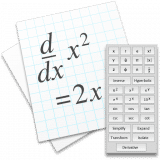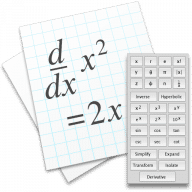Equation Calculator
1.1
5.0
0.0
Equation Calculator# Equation Calculator for Mac1.1

13 October 2011

Scientific calculator with algebra, calculus and numeric computation.

## What is Equation Calculator for Mac

Equation Calculator is a scientific calculator which does symbolic and algebraic manipulation, algebra and calculus as well as numeric computation. You can define variables and functions, evaluate symbolic derivatives, numeric integrals and matrix operations.

## What's new in Equation Calculator

Version 1.1:
• Users can now type text comments in documents.
Try our new feature and write a detailed review about Equation Calculator. All reviews will be posted soon.
Write your thoughts in our old-fashioned comment
MacUpdate Comment Policy. We strongly recommend leaving comments, however comments with abusive words, bullying, personal attacks of any type will be moderated.
0.0
(0 Reviews of )
There are no reviews yet
Help the community
There are no reviews yet, be the first to leave one